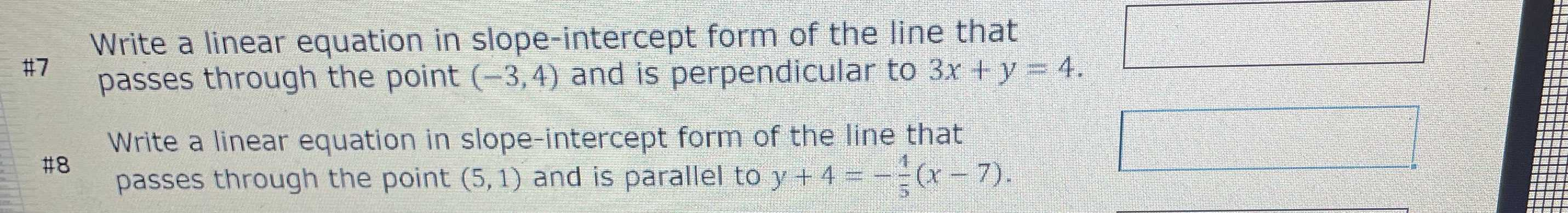### ¿Todavía tienes preguntas de matemáticas?

Pregunte a nuestros tutores expertos
Algebra
Pregunta$$\# 7$$ Write a linear equation in slope-intercept form of the line that passes through the point $$( - 3,4 )$$ and is perpendicular to $$3 x + y = 4 .$$

$$\# 8$$ Write a linear equation in slope-intercept form of the line that passes through the point $$( 5,1 )$$ and is parallel to $$y + 4 = - \frac { 1 } { 5 } ( x - 7 )$$